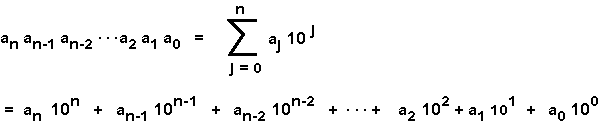```7305 = 7 ×  103  +  3 × 102  +  0 × 101  +  5 ×  100
```

# Decimal Notation

For base 10 representation (often called decimal representation), the rules of positional notation are:

1. The base is 10.
2. There are 10 digits: 0, 1, 2, 3, 4, 5, 6, 7, 8, 9 .
3. Positions correspond to integer powers of 10, starting with power 0 at the rightmost digit, and increasing right to left.
4. The digit placed at a position shows how many times that power of 10 is included in the number.

A compact way of writing this is:Any integer can serve as the base for a positional representation system. Five can serve as a base.

### QUESTION 7:

Here are the rules for positional notation. Fill in the blanks to work with base five:

1. The base is .
2. There are "digits": , , , , .
3. Positions correspond to integer powers of , starting with power at the rightmost digit, and increasing right to left.
4. The digit placed at a position shows how many times that power of included in the number.